Choose Language Hide Translation Bar
Highlighted

## Update X-Axis while grouping in Graph Builder

Hi Everyone,

I have a query. If I have a table like below. If I plot TIME on X-axis and group the X-axis by TYPE. It will shows all five values on X-Axis for both groups, even if their is no data for that. It unnecessarily using the graph space. One way is I can nest the X-axis(TIME) with column TYPE but this works only when both columns are of type nominal. Is their a way if both columns are of different type?

Thanks

7 REPLIES 7
Highlighted

## Re: Update X-Axis while grouping in Graph Builder

Using the GraphBuilder interface, right click on the column Time, and select Formula and enter this formula Format( :TIME, "m/d/y" ) .  This will now appear in the column selector as Transform[Time]. Drag this to X, then drag  column Type so that Time is nested within Type.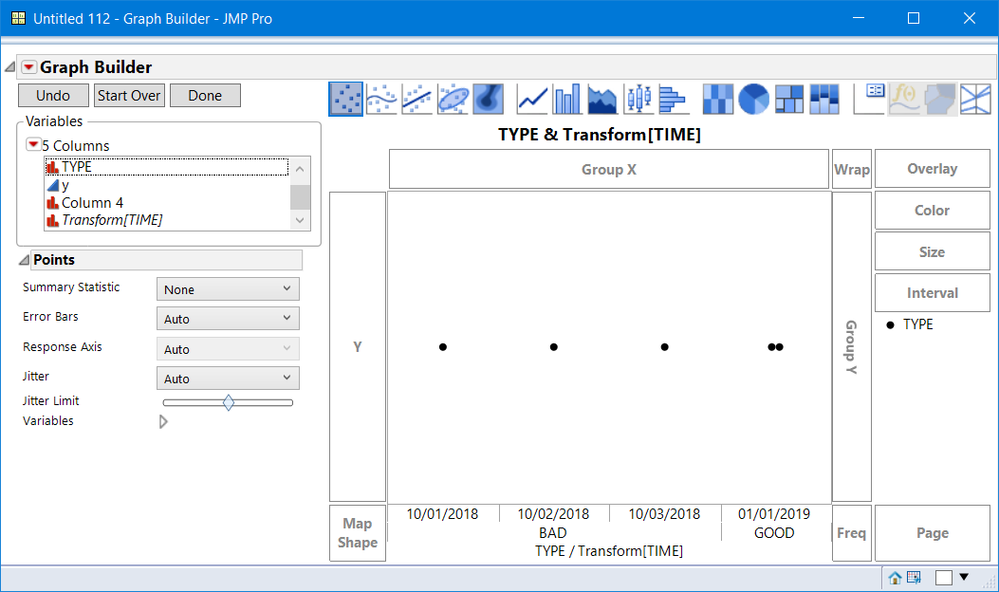Highlighted

## Re: Update X-Axis while grouping in Graph Builder

@gzmorgan0,
This solution is only applicable when both column are of nominal type but I have one column of nominal modeling type and other column of Continuous modeling type.
Highlighted

## Re: Update X-Axis while grouping in Graph Builder

Do you have an example where this does not work?  When you transform the time to character, by default charater is nominal.  Your response does not make sense to me.

Highlighted

## Re: Update X-Axis while grouping in Graph Builder

You can take the same example, I posted. Here column "TIME" is of modeling type Continuous and column "TYPE" is of modelling type Nominal. These two columns you can't nest these two columns in graph builder.
Highlighted

## Re: Update X-Axis while grouping in Graph Builder

You must not have understood my response:

• Transform Time to character,
• Use the transformed column in the nesting.

The embedded graph in my post used your example, with a random Y variable.

Highlighted

## Re: Update X-Axis while grouping in Graph Builder

This works for this case only. Say if, I have column "TIME(Sec)" it measures the time for a particular process and it is a continuous variable and I don't want to change to nominal then how I will plot plot similar graph between Time and type.
Highlighted

## Re: Update X-Axis while grouping in Graph Builder

JMP platforms work as they are scripted by the JMP developers. You can make a Wish List change and maybe it will change in the future,

I do not know how to make GraphBuilder do what I think you want unless you restructure your data table. GraphBuilder will not nest different data types, and X-Grouping uses the same X scale.  So the only way to do this is to make each group's (Type) time (X variable) its own column and drag them side by side.  Below is a capture of your table layout and a restructured, Split table: Split  :Time by :Type grouping by :row and keeping all columns.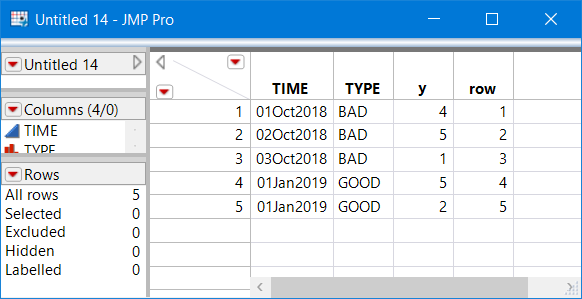Your specified table layout - Row # column added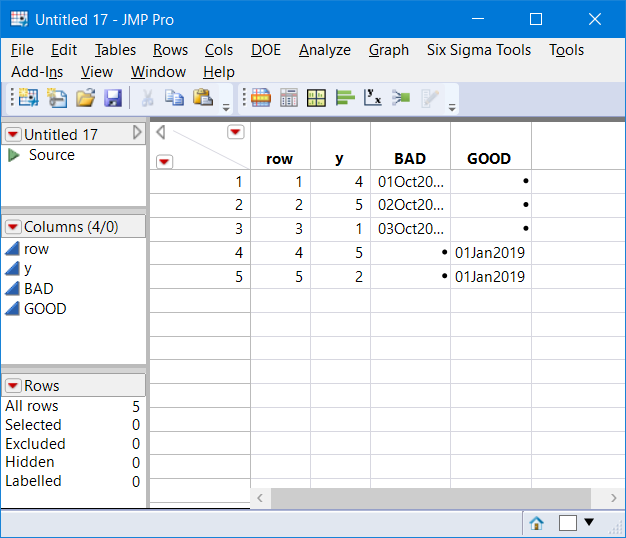Date Split by Type, Grouped by row #

Below is the side-by-side GraphBuilder created with the split table time table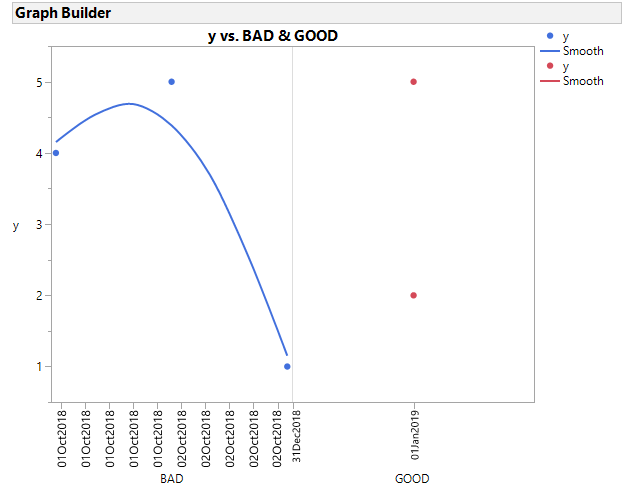Here is the script.

``````Graph Builder(
Variables( X( :BAD ), X( :GOOD ), Y( :y ) ),
Elements(
Position( 1, 1 ),
Points( X, Y, Legend( 19 ) ),
Smoother( X, Y, Legend( 20 ) )
),
Elements(
Position( 2, 1 ),
Points( X, Y, Legend( 21 ) ),
Smoother( X, Y, Legend( 22 ) )
)
)``````

Of course you do not need to restructure, you could create new variables via the transform, a new variable for each type then drag the transfomed variables to the X-Axis, side-by-side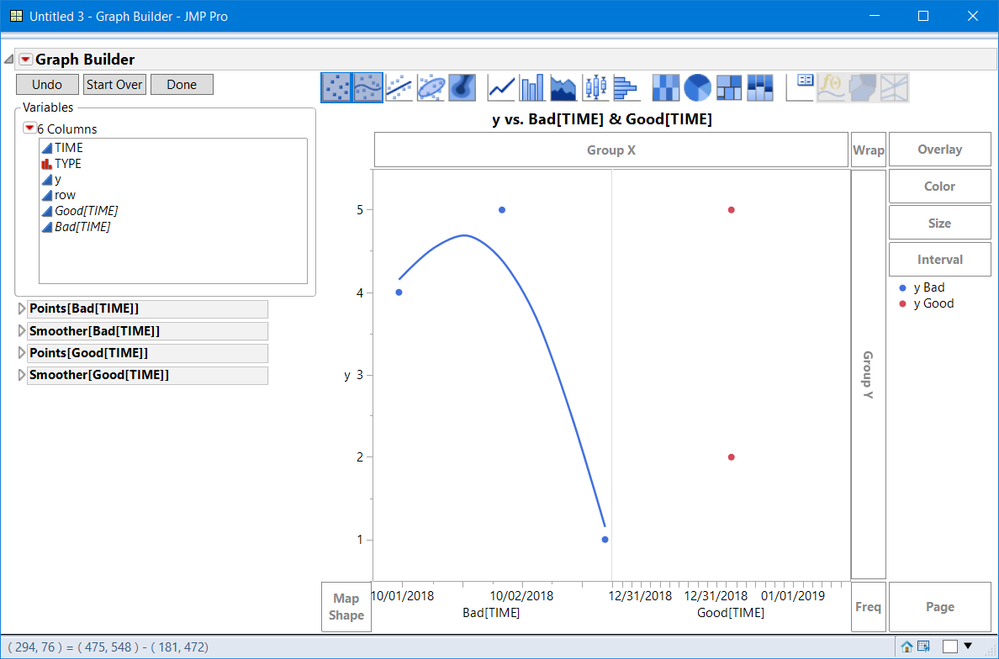Below is the saved script. Splitting the table will be the easiest, if there are many groups

``````Graph Builder(
Size( 856, 534 ),
Show Control Panel( 0 ),
Variables(
X( Transform Column( "Bad[TIME]", Formula( If( :TYPE == "BAD", :TIME ) ) ) ),
X(
Transform Column(
"Good[TIME]",
Formula( If( :TYPE == "GOOD", :TIME ) )
)
),
Y( :y )
),
Elements(
Position( 1, 1 ),
Points( X, Y, Legend( 17 ) ),
Smoother( X, Y, Legend( 18 ) )
),
Elements(
Position( 2, 1 ),
Points( X, Y, Legend( 19 ) ),
Smoother( X, Y, Legend( 20 ) )
),
SendToReport(
Dispatch( {}, "Bad[TIME]", ScaleBox, {Format( "m/d/y", 10 )} ),
Dispatch(
{},
"Good[TIME]",
ScaleBox,
{Format( "m/d/y", 10 ), Min( 3629059200 ), Max( 3629232000 ),
Interval( "Numeric" ), Inc( 17.28 ), Minor Ticks( 1 )}
),
Dispatch(
{},
"400",
ScaleBox,
{Legend Model( 17, Level Name( 0, "y Bad", Item ID( "y", 1 ) ) ),
Legend Model( 19, Level Name( 0, "y Good", Item ID( "y", 1 ) ) )}
),
Dispatch(
{},
"400",
LegendBox,
{Legend Position( {17, , 18, [-1], 19, , 20, [-1]} ),
Position( {0, -1, 1, -1} )}
)
)
)``````
Article Labels

There are no labels assigned to this post.